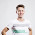## Saturday, 30 July 2016

### Super ASCII String Checker

Problem Statement:

In the Byteland country a string "S" is said to super ascii string if and only if count of each character in the string is equal to its ascii value.

In the Byteland country ascii code of 'a' is 1, 'b' is 2 ...'z' is 26.

For eg1 - String "bba" is super ascii string
Explanation:- .String "bba"
The count of character 'b' is 2. Ascii value of 'b' is also 2.
The count of character 'a' is 1. Ascii value of 'a' is also 1.
Hence string "bba" is super ascii string.

For eg2 - String "acc" is not super ascii string
Explanation:- String "acc"
The count of character 'a' is 1. Ascii value of 'a' is also 1
The count of character 'c' is 2. But the ascii value of 'c' is 3
Hence string "acc" is not super ascii string.

Program:

import java.util.*;
class PS
{
public static void main(String sap[])
{
int i,j;
Scanner sc = new Scanner(System.in);

//taking input string
System.out.print("\nEnter String: ");
String str = sc.next();

//character array for retriving the value of respective character
char char_arr[] = new char;

int char_count=97;
for(i=1;i<27;i++)
{
char_arr[i] = (char)(char_count++);
}

//it would act like a hash table
int result_arr[] = new int;

for(i=1;i<27;i++)
result_arr[i]=0;

for(i=0;i<str.length();i++)
{
for(j=1;j<27;j++)
{
if(char_arr[j]==str.charAt(i))
{
int temp = result_arr[j];
result_arr[j]=temp+1;
break;
}
}
}

//comparing result array with its index if yes then accept else break
for(i=1;i<27;i++)
{
if(result_arr[i]>0)
{
if(result_arr[i]!=i)
{
break;
}
}
}

//if value of 'i' would be less then 27 then string is rejected...
if(i<27)
System.out.print("\n"+str+" not a super ascii string...");
else
System.out.print("\n"+str+" is super ascii string...");
}
}

Output:

1. Enter String: bab
bab is super ascii string...

2. Enter String: ccdddd
ccdddd not a super ascii string...

1.A little bit complicated code for me, cause I have learned Java only for a few days, but my friend said that after reading this service about parseint java https://explainjava.com/convert-string-int-java/ I will understand everything without any extra problems.

2.import java.util.*;
public class superascii {
public static void main(String args[])
{
Scanner n=new Scanner(System.in);
String a=n.next();
HashSet hs=new HashSet();
for(int i=0;i arr=new ArrayList();
String rep="";
for(String ar:arr)
{
rep+=ar;
}
int count[]=new int[hs.size()];
char c[]=new char[hs.size()];
for(int i=0;i<hs.size();i++)
{
c[i]=rep.charAt(i);
}
for(int i=0;i<hs.size();i++)
{
count[i]=a.length()-a.replaceAll(arr.get(i),"").length();
}
String out="NO";
for(int i=0;i<hs.size();i++)
{
if(count[i]==(int)c[i]-96)
{
out="YES";
}
else
{
out="NO";
break;
}
}
System.out.println(out);
}
}